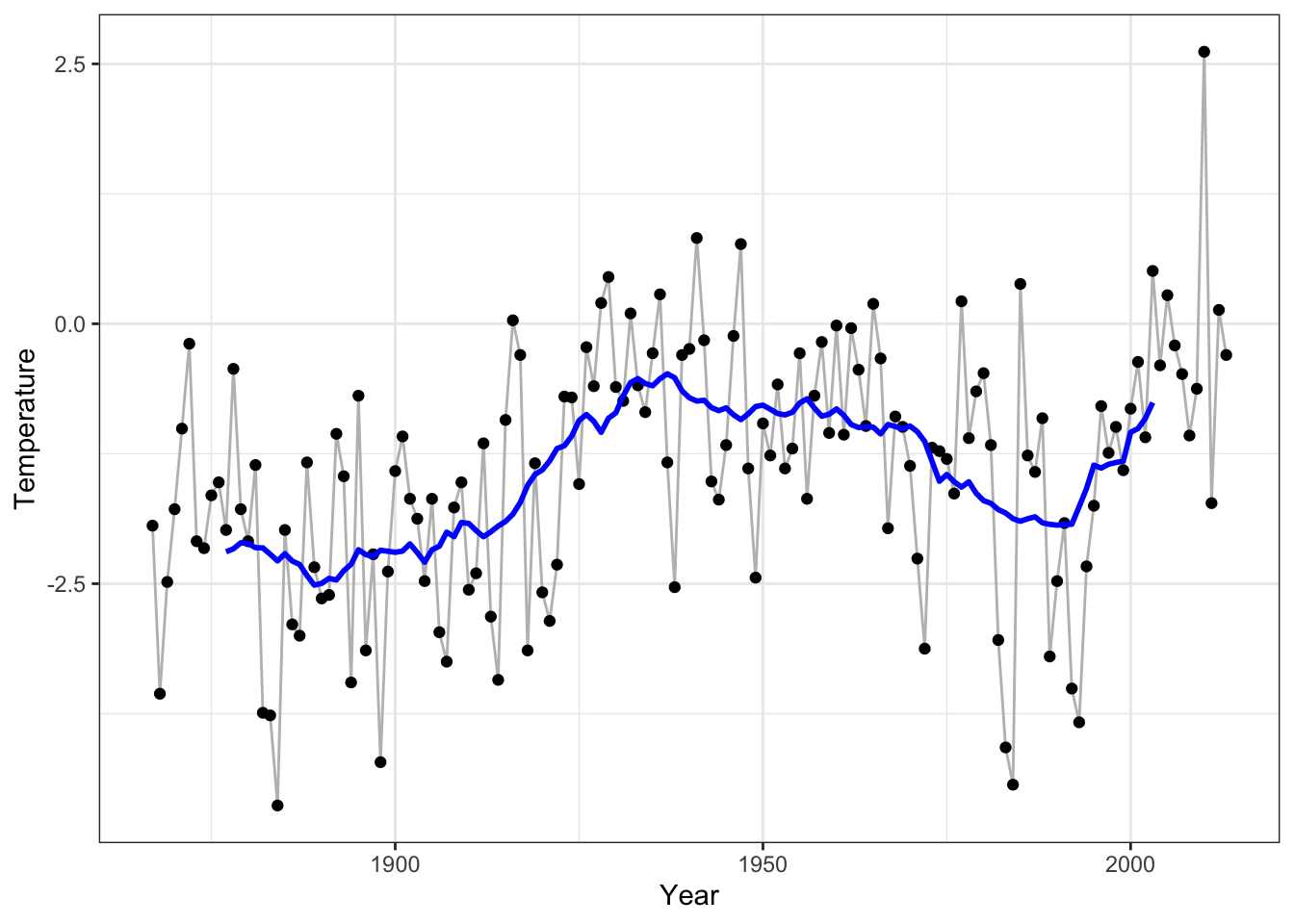# Chapter 3 Bivariate smoothing

The focus of this chapter is on estimating how one variable, $$Y$$, is smoothly related to another, $$X$$. Thus we are directly aiming for an estimate of (aspects of) the conditional distribution of $$Y$$ given $$X$$. If both variables are real valued, we can get a pretty good idea of their relation by simply looking at a scatter plot, and what we are aiming for is also often referred to as scatter plot smoothing. In some cases $$X$$ represents a random variable, while in other cases, as the temperature example below, $$X$$ represents a deterministic variable. In the example below $$X$$ is time, and in other applications $$X$$ could be fixed by an experimental design.

One of the examples that will be used throughout is the monthly and yearly temperatures in Nuuk, Greenland, see Vinther et al. (2006). The updated data is available from the site SW Greenland temperature data.

p_Nuuk <- ggplot(Nuuk_year, aes(x = Year, y = Temperature)) + geom_point()
p_Nuuk + geom_smooth(se = FALSE) +
geom_smooth(
method = "lm",
formula = y ~ poly(x, 10),      # A degree-10 polynomial expansion
color = "red",
se = FALSE
) +
geom_smooth(
method = "gam",
formula = y ~ s(x, bs = "cr"),   # A spline smoother via 'mgcv::gam()'
color = "purple",
se = FALSE
)

ggplot(Nuuk, aes(x = Month, y = Temperature)) +
geom_line(aes(group = Year), alpha = 0.3) +
geom_point(alpha = 0.3) +
geom_smooth(color = "red", se = FALSE)  # A spline smootherFigure 3.1: Nuuk average yearly temperature in degrees Celsius (left) smoothed using loess (black), a degree 10 polynomial (red) and a smooth spline (purple). Nuuk annual temperature cycles (right) smoothed using a spline.

### References

Vinther, B. M., K. K. Andersen, P. D. Jones, K. R. Briffa, and J. Cappelen. 2006. “Extending Greenland Temperature Records into the Late Eighteenth Century.” Journal of Geophysical Research: Atmospheres 111 (D11).